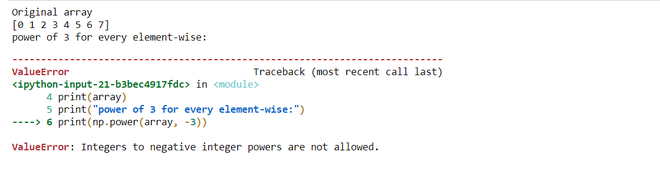Open In App

# Get the powers of a NumPy array values element-wise

NumPy is a powerful N-dimensional array object and its use in linear algebra, Fourier transform, and random number capabilities. It provides an array object much faster than traditional Python lists. numpy.power() is used to calculate the power of elements. It treats first array elements raised to powers from the second array, element-wise.

Syntax: numpy.power(arr1, arr2, out = None, where = True, casting = ‘same_kind’, order = ‘K’, dtype = None)

Parameters :

arr1     : [array_like]Input array or object which works as base.
arr2     : [array_like]Input array or object which works as exponent.
out      : [ndarray, optional]Output array with same dimensions as Input array,
placed with result.
**kwargs : Allows you to pass keyword variable length of argument to a function.
It is used when we want to handle named argument in a function.
where    : [array_like, optional]True value means to calculate the universal
functions(ufunc) at that position, False value means to leave the
value in the output alone.

So, let’s discuss some examples related to the getting power of an array.

Example 1: compute the power of an array with different element-Wise values.

## Python3

 `# import required modules``import` `numpy as np`  `# creating the array``sample_array1 ``=` `np.arange(``5``)``sample_array2 ``=` `np.arange(``0``, ``10``, ``2``)` `print``(``"Original array "``)``print``(``"array1 "``, sample_array1)``print``(``"array2 "``, sample_array2)` `# calculating element-wise power``power_array ``=` `np.power(sample_array1, sample_array2)` `print``(``"power to the array1 and array 2 : "``, power_array)`

Output:

```Original array
array1  [0 1 2 3 4]
array2  [0 2 4 6 8]
power to the array1 and array 2 :  [    1     1    16   729 65536]```

Examples 2: computing the same power for every element in the array.

## Python3

 `# import required module``import` `numpy as np`  `# creating the array``array ``=` `np.arange(``8``)``print``(``"Original array"``)``print``(array)` `# computing the power of array``print``(``"power of 3 for every element-wise:"``)``print``(np.power(array, ``3``))`

Output:

```Original array
[0 1 2 3 4 5 6 7]
power of 3 for every element-wise:
[  0   1   8  27  64 125 216 343]```

Examples 3: computing the power of decimal value.

## Python3

 `# import required modules``import` `numpy as np`  `# creating the array``sample_array1 ``=` `np.arange(``5``)` `# initialization the decimal number``sample_array2 ``=` `[``1.0``, ``2.0``, ``3.0``, ``3.0``, ``2.0``]` `print``(``"Original array "``)``print``(``"array1 "``, sample_array1)``print``(``"array2 "``, sample_array2)` `# calculating element-wise power``power_array ``=` `np.power(sample_array1, sample_array2)` `print``(``"power to the array1 and array 2 : "``, power_array)`

Output:

```Original array
array1  [0 1 2 3 4]
array2  [1.0, 2.0, 3.0, 3.0, 2.0]
power to the array1 and array 2 :  [ 0.  1.  8. 27. 16.]```

Note: you can not compute negative power

Example 4:

## Python3

 `# importing module``import` `numpy as np`  `# creating the array``array ``=` `np.arange(``8``)``print``(``"Original array"``)``print``(array)``print``(``"power of 3 for every element-wise:"``)` `# computing the negative power element``print``(np.power(array, ``-``3``))`

Output: